FLAC3D Modeling • Tutorials

# Tutorial: Illustrative Model — Mechanics of Using FLAC3D

This first tutorial provides the new user an initial look at the basics of modeling with FLAC3D. The structure of this tutorial, delineated below, corresponds to the general structure for FLAC3D modeling that is presented at length in the sections of the Problem Solving with FLAC3D section and is charted in General Solution Procedure, Illustrated.

This tutorial problem presents a trench excavated in a soil with low cohesion. After failure is observed during excavation, support is added to stabilize the trench.For this tutorial, the sequence charted in General Solution Procedure, Illustrated may be broken down as follows.

First, a model must be set up in FLAC3D, which comprises the first seven steps below.

1. Create a project for the model.

The project acts as a central collection of all resources and outputs that will be part of the FLAC3D model.

2. Create a mesh that discretizes the model volume.

The grid defines the geometry of the problem.

3. Assign names to regions of the model by using Groups.

Groups are used to label areas of specific interest in the model.

4. Assign constitutive behavior and material properties.

The constitutive behavior and associated material properties dictate the type of response the model will display upon disturbance (e.g., deformational response due to excavation).

5. Apply boundary conditions.

Initial conditions define the in-situ state (i.e., the condition before a change or disturbance in the problem state is introduced).

7. Step to initial equilibrium state.

After the model setup is complete, the initial equilibrium state is calculated for the model.

Next, the model is ready for alteration, perturbation, or other examination. This is usually the point where the intended analysis can really begin. The next steps shown are ones that might be looped or branched in many variations, depending on the needs of the analysis.

1. Alteration of the model as required by the problem.

Alteration(s) are then made (excavate material, change boundary conditions, install support, etc.), and the resulting response of the model is calculated. At this point, the nature of the problem will determine what steps will follow and there is no specific normative approach. In this tutorial, we continue and conclude with the following steps.

2. Examine model response (plotting).

3. Further alteration (e.g., install support).

4. Project completion (storage).

Solutions

The actual solution of the problem is different for an explicit program like FLAC3D than it is for conventional implicit-solution programs. (This is a large topic; see the FLAC3D Theory and Background section.) FLAC3D uses an explicit time-marching method to solve the discretized equations. The solution is reached after a series of computational steps. In FLAC3D, the number of steps required to reach a solution can be controlled automatically by the code or manually by the user. However, the user ultimately must determine if the number of steps is sufficient to reach convergence to equilibrium. In this tutorial, the way this is done is described in the Step to Equilibrium and Further Discussion: Step to Equilibrium sections below.

Building a Data File

FLAC3D is a command-driven program. Those commands can be issued from the command prompt, or, in a number of cases, they are generated interactively by the user interface. There is no difference between the commands as issued from one place or the other, merely the matter of efficiency and speed in the issuing, and user preference.

However, there is a third place where commands are saved/recorded: the data file. The data file is essentially a batch file, that is, a list of commands that can be issued and processed in unbroken sequence. This is both more efficient than either the command prompt or mouse/keyboard commands—either of which is limited to issuance of one command at a time—and has the added advantage of being able to provide a readable representation of a problem from beginning to end.

Model construction in FLAC3D is thus a matter of data file construction. Though typing a data file in its entirety prior to any command processing is possible, in practice this is rarely done. Instead, a final data file is arrived at in a stepwise fashion that is usually a blend of commands from the file itself and/or other data files, the command prompt, and the user interface, not to mention the number of “drafts” that occur in refining the modeling approach taken to best represent the problem. The art of data file construction in FLAC3D is a matter of efficiently using all three command sources, as well as the save/restore system, to work through the problem.

The State Record Pane

In FLAC3D, all operations that modify the current model state do so by issuing a command. Operations that only affect the program or project state do not have this requirement and generally do not issue a command in the interface, although commands exist that only change those states. All commands that occur in a given model state are recorded in the State Record. This record is included in save files that represent the model state created using model save. Commands are recorded regardless of whether they are generated directly by the user or interactively by the user interface. These commands are available for review in the State Record Pane. This state record can be played back directly using the program playback command, or they can be converted into an equivalent data file for later repetition or parameterization.

The state record does not record the contents of a data file that is executed. These commands are considered to be already recorded in the data file itself. Rather, the record tracks the data file name, size, and last modified stamp for reference.

## Create a New Project

Create a project for this tutorial.

1. Choose File ‣ New Project… from the main menu.
2. Navigate to the folder where the project file will be saved.
3. Name the project “tutorial1” in the File Name field.
4. press or press Enter .Set Up the Workspace

Setup and save the workspace.

1. Select Layout ‣ Wide .
2. Select Panes ‣ State Record .
3. Select File ‣ Save Project .

When the project is saved in step 3, the state of the workspace (including the current layout settings) is saved with the project. This tutorial will make use of tools available in the State Record and Model panes, so we will need them both at hand.

Create a Data File

For this tutorial, a single complete data file for the problem will be created.

1. Select File ‣ Add New Data File… or press Ctrl + N.
2. At the “File Name” field, enter “tutorial”.
3. Press or press Enter .

Note the new data file is made the active pane.

Next: Generate the Grid

Discussion

When a project file is created and saved, FLAC3D uses the folder containing that project file as the current working directory. This location will be the default for most file-managing dialogs created by the user-interface (although some may remember the location of the last file accessed in that session). In addition, any command that saves a file may be issued without a path, as the working directory is assumed if no path is supplied.

Creating a project at the outset of any modeling process is the strongly recommended approach to using FLAC3D. The project handles a range of tasks for managing the modeling project, in addition to the ones described above. The existence of a project makes retaining settings, re-opening or restoring files, and project support and archiving significantly easier.

Refer to the Projects section for complete information on working with projects.

### In the Interface

After creating the project and data file, some additional aspects of the program’s behavior may be noted.

• The FLAC3D program title bar shows the project name. It also reports the full version of the program as well.• The data file has been added to the Project pane (be sure you are using the “Wide” layout; select Layout ‣ Wide if your FLAC3D does not match the image shown).
• Also note that when the data file is created, a blank data file is opened in an Edit pane, which is stacked in a tab set on top of the Plot01 pane and automatically made the active pane (the title bar of the active pane in the program is colored a darker blue than those of the inactive panes). In general, creating a new file (or plot, or other program resource) will make the pane containing that resource the active pane.
• The name of the data file appears at the top of the Edit pane containing the file.
• The Control Panel and the program toolbar contain tools for working in the Edit pane. To further illustrate, select the Plot01 tab adjacent to the tutorial tab and see how the Control Panel and toolbar change. Next click the title bar of the Console pane, then click the title of the Project pane. Note the changes, and that in each case the clicked item becomes the active pane. At all times, the Control Panel and the program toolbar will contextually supply tools/facilitites based on the pane type that is active.

## Generate the Grid

The grid for this problem is created with one command.

1. Type the lines below into the “tutorial.dat” data file (or you can cut and paste them directly from the listing below).
; create geometry/zones
model new
point 0 (0,0,0) point 1 (10,0,0) ...
point 2 (0,10,0) point 3 (0,0,10) ...
size 3,5,5,7     ...
ratio 1,1,1,1.5  ...
dim 1 4 2 fill group "exc"
model save "geom"

1. Press the Execute button (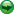). The commands are processed and echoed in the Console pane output.
2. Activate the Model pane (click the tab that says “Model”).
3. Right-click and drag on the model to 3D rotate. See View Manipulation for more ways of manipulating the view if you’d like to go further in examining the model.

Previous: Create a New Project | Next: Create Model Groups

Discussion

The first line clears FLAC3D of model-state information (see model new for reference). To keep things simple, we will re-run the entire data file usingeach time we add lines as this tutorial progresses. Putting model new at the start ensures the model will run without error each time we do so.

The second line creates the geometry. In that line, a radial-brick primitive is used to create zones. The keywords that follow the name of the primitive specify size, grid spacing (ratio), and brick-size (dimensions). This command occupies six typed lines of text, but through use of the continuation operator ( … ) FLAC3D treats these as one line, making it a single command.

Creating a problem’s grid with primitives using the zone create command is a fast and efficient method of constructing zones. However, as the complexity of model geometry increases, the use of primitives also gets increasingly difficult, and FLAC3D offers other methods of constructing grids that may be preferable. An introduction to zone construction methods and the multiple considerations that go into grid construction are described in Grid Generation.

It is a recommended practice to save the model state at the point where the zones for the problem geometry are created. Since one zone create command is sufficient to obtain the geometry needed for this problem, the final line above saves the model state in the file “geom.f3sav” (note the file extension is added automatically).

Some elaboration and additional examples of meshing with primitives follows in Further Discussion: Meshing With Primitives.

Observations about the program response to the commands issued here is covered in the topic In the Interface.

### Further Discussion: Meshing With Primitives

One basic method of grid generation is performed via the zone create command and its associated keywords, which defines the number of zones in a model and shapes the grid to fit a specified problem region.

Several primitive shapes are built into the generator to expedite mesh generation for simple problem shapes. These include brick, wedge, pyramid, and cylinder shapes. The example listing below is the command used in Tutorial: Working with FISH to create a brick-shaped grid containing six zones in the $$x$$-direction, eight zones in the $$y$$-direction, and eight zones in the $$z$$-direction.

model new
zone create brick size 6,8,8


The number of zones is specified by the size keyword.

Be careful when selecting the number of zones for a model, because a balance must be struck between the accuracy required and the solution speed.

Although there are many aspects of a FLAC3D model that affect the calculation speed, once the basic parameters are defined, the speed varies directly as a function of the number of elements. As a rule-of-thumb, models containing up to roughly 5000 elements will typically reach a solution state for a given alteration in approximately 2000 to 4000 steps. On a 2.67 GHz Intel i7 computer, the runtime for a 5000 element model to perform 4000 steps is roughly 30 seconds. Check the speed of calculation on your computer for the specific model to estimate the runtime required.

When building a FLAC3D model, it is a good practice to start with a grid that has fewer zones to perform simple test runs and make refinements to the grid. Then, increase the number of zones to improve the accuracy.

In its simplest form, the zone create command can supply new coordinates to a grid. For example, assume that the actual coordinates of the trench model run from -10.0 to 10.0 in the $$x$$-direction, -10.0 to 10.0 in the $$y$$-direction, and -20.0 to 0.0 in the (vertical) $$z$$-direction. The next listing shows how this would be specified.

model new
zone create brick size 6,8,8 ...
point 0 -10, -10, -20 ...
point 1  10, -10, -20 ...
point 2 -10,  10, -20 ...
point 3 -10, -10,   0


In the preceding example, point 0, point 1, point 2, and point 3 are keywords that denote four of the corners of a standard FLAC3D mesh (see diagram below). Notice that an ellipsis (…) is given at the end of the first, second, third, and fourth lines. The ellipsis denotes that the next line is a continuation of the same command, consequently the first command, zone create, appears on five typed lines in the text but is processed by FLAC3D as a single line.Figure 1: Corner coordinate keywords for a standard FLAC3D brick mesh shape.

Only four corners are required to define a parallelepiped-shaped mesh. More corners can be specified to define an irregular surface. The next example shows how to make a sloping surface at the top of the mesh.

model new
zone create brick size 6,8,8 ...
point 0 -10, -10, -20 ...
point 1  10, -10, -20  point 2 -10,  10, -20 ...
point 3 -10, -10,   0  point 4  10,  10, -20 ...
point 5 -10,  10,  10  point 6  10, -10,   0 ...
point 7  10,  10,  10


The coordinates corresponding to the keywords point 0, point 1, point 2, point 3, point 4, point 5, point 6, and point 7 can be located at arbitrary points in space. However, the eight corners must have the topological sense shown in the diagram above (i.e., point 0, point 1, point 2, point 3 must form a right-handed system) to create positive zone volumes. Non-coplanar faces are permitted, but they should be avoided if possible because a small volume error may be introduced.Figure 2: The simple brick and the sloped-surface brick from the preceding two examples.

Note the boundaries of the model influence the results. preceding statement swapped in for following flagged statement, which is flagged pending the fate of the use of common commands tutorial In :ref:basiccli it is noted that the boundaries of the model influence the results (see :flag2:figure reference tbd). The boundary must be placed far enough away from the excavation to reduce these effects. A gradually graded mesh can be created in FLAC3D to move the model boundaries farther out without significantly increasing the number of zones. For example, the command zone create radial-brick creates a radially graded mesh around a brick-shaped mesh. The command in the next example creates a 3 × 5 × 5 zone brick-shaped mesh surrounded by a seven-zone radially graded mesh.

model new
point 0 (0,0,0) point 1 (10,0,0) point 2 (0,10,0) point 3 (0,0,10) ...
size 3,5,5,7 ...
ratio 1,1,1,1.5 ...
dim 1,4,2   fill


The $$x$$, $$y$$, $$z$$ coordinate system in FLAC3D is always right-handed and, as a default, the $$z$$-axis is drawn in the vertical direction on the screen. In the simple brick examples above, we assumed the $$z$$-axis was pointing in the vertical direction. However, we do not have to interpret the $$z$$-axis to mean the up direction. For this one, we will assume that the $$y$$-axis is the vertical direction. As long as we define the model in a right-handed system, we can create the grid in any direction we desire.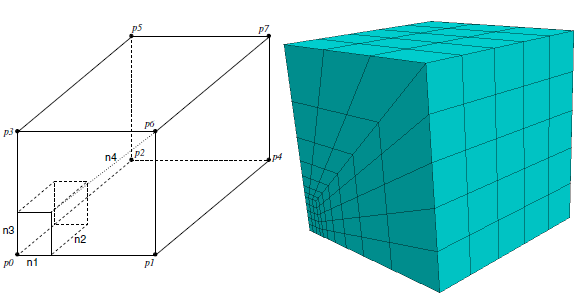Figure 3: (Left) Element numbering for a radially graded mesh around brick (size n1 n2 n3 n4); (Right) the grid resulting from the previous example.

The size keyword specifies the number of zones in the brick, and the number radially surrounding the brick (as seen in the resulting grid on the right). The keyword ratio is given to manipulate the grid spacing. The four values that follow ratio are geometric ratios between successive zone sizes. The first three values are ratios for the zones in the brick, and the fourth value is the ratio for the zones surrounding the brick. In this example, the ratio is 1.0 for the brick zones and 1.5 for the zones surrounding the brick. This causes each successive ring of zones surrounding the brick to be 1.5 times larger in the radial direction. The dimension keyword defines the dimensions of the brick region (i.e., 1 m × 4 m × 2 m). The fill keyword fills the brick region with zones. If fill is omitted, no zones will be generated within the brick region.

Several zone create radial-brick commands can be given to create different regions of a model. For example, the preceding example creates one-quarter of the grid for the trench model in this tutorial. The following example shows how four zone create radial-brick commands that create quarter symmetries may be combined to create an entire grid for the problem.

model new
point 0 (0,0,0)  point 1 (10,0,0)  ...
point 2 (0,10,0)  point 3 (0,0,10) ...
size 3 5 5 7                                      ...
rat 1 1 1 1.5                                     ...
dim 1 4 2 fill
point 0 (0,0,0)  point 1 (0,0,10)   ...
point 2 (0,10,0)  point 3 (-10,0,0) ...
size 5 5 3 7                                       ...
rat 1 1 1 1.5                                      ...
dim 2 4 1 fill
point 0 (0,0,0)  point 1 (-10,0,0)  ...
point 2 (0,10,0)  point 3 (0,0,-10) ...
size 3 5 5 7                                        ...
rat 1 1 1 1.5                                       ...
dim 1 4 2 fill
point 0 (0,0,0)  point 1 (0,0,-10) ...
point 2 (0,10,0)  point 3 (10,0,0) ...
size 5 5 3 7                                       ...
rat 1 1 1 1.5                                      ...
dim 2 4 1 fill


Notice that size, ratio, and dimension all refer to local axes as defined by point 0, point 1, point 2, point 3 in the radial-brick diagram above, not to the global $$x$$, $$y$$, $$z$$-axes. The values will change, depending on the specification of point 0, point 1, point 2, and point 3. The radially graded trench model is shown in the next figure. Be sure that all gridpoints match at boundaries between shapes. Errors will occur in your analysis if gridpoints of connected shapes are not at the same locations along boundaries. Refer to Grid Generation for further discussion on the creation of meshes and the proximity tolerances of adjacent gridpoints.

The preceding example illustrates the requirement of accurate mapping of adjacent elements in FLAC3D. There are other ways to achieve the same result shown above, because we can take advantage of planes of symmetry. Parts of the model can be reflected about specified planes to produce a larger model. The following example shows how this can be achieved.

model new
point 0 (0,0,0)  point 1 (10,0,0)  ...
point 2 (0,10,0)  point 3 (0,0,10)  ...
size  3,5,5,7   ...
ratio 1,1,1,1.5 ...
dim 1 4 2 fill
zone reflect dip  0 dip-direction 90
zone reflect dip 90 dip-direction 90


The meshes resulting from the preceding two examples are the same. However, looking at “Plot01”, one difference may be observed: in the first example, four zone groups are created (as seen in the figure above), while in the second, only one zone group is created. This is because FLAC3D will automatically group all the zones that are generated from the operation of a zone create command.

When selecting a value for the geometric ratio, there are a number of criteria that should be considered.

• Finer meshes lead to more accurate results in that they provide a better representation of high stress gradients.
• Accuracy increases as zone aspect ratios tend to unity.
• If different zone sizes are needed, a more gradual change from the smallest to the largest will improve the results; abrupt changes in zone size should be avoided.

However, as the mesh is made finer and the number of zones increases, more RAM is required and the computation time lengthens. Improving the mesh geometry by adjusting the zone size will certainly improve RAM efficiency, and will reduce computation time. It will also take some time to plan and set up. Moving from a “brute force” approach (i.e., single-sized elements in a large uniform mesh) to a more elegant solution will improve RAM use and calculation speed when dealing with a more complicated geometry. Grid Generation presents an overview of the methods of grid generation available in FLAC3D.

### In the Interface

After processing the commands that generate the problem mesh, some aspects of the interface can be observed.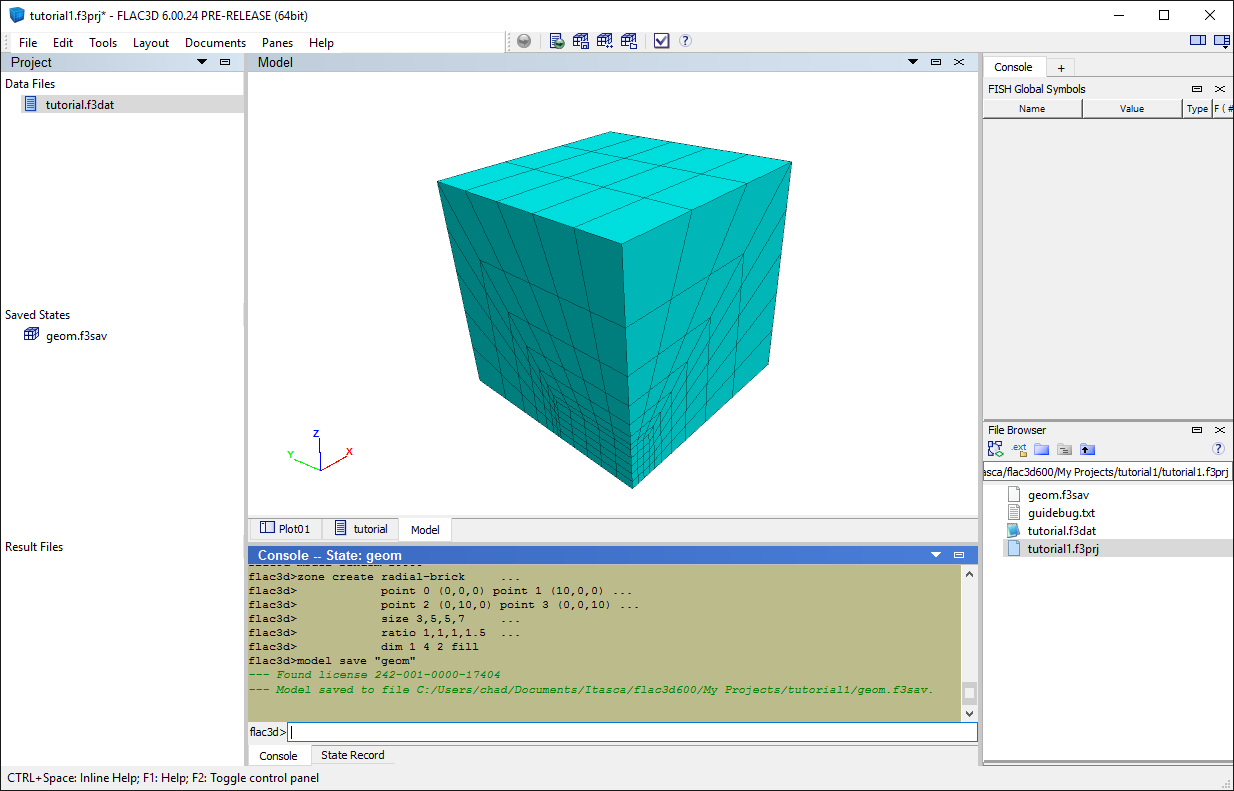• An asterisk (*) appears next to the project name in the title bar to indicate the project is not saved. In FLAC3D, when an asterisk appears adjacent to a name in a list or on a title bar, it indicates that the item has been modified since it was last saved.
• The “tutorial.dat” file does not have an asterisk next to it. This is because the file was automatically saved when the Execute button () was pressed—which is the normal program response when that button is used.
• The Project pane now lists “geom.f3sav” in the “Saved States” list.
• The Model pane displays the model zones that are created.
• Click on the title bar of the Project pane, then click the title bar or the tab for the Model pane, then the Console pane. When clicked, the title bar of the clicked pane turns darker blue to indicate it is now the active pane. Observe the effect of changing the active pane on the toolbar and the Control Panel —tracking what pane in the program is active is important, since the toolbar and the Control Panel supply facilities that are specific to that pane type.
• The commands that were processed from the data file when the Execute button () was pressed are echoed in the console output. The observant will note that the effect of pressing the button is really to cause a program call command to be issued for the currently active data file.

## Create Model Groups

Next we obtain zone face groups that will be used later when setting up boundary and initial conditions. In addition, we create the five excavation stages by grouping the zones that will be removed from the model at each stage.

1. Make the Model pane active, if it isn’t already (click “Model” tab).
2. Press the Auto Assign Face Groups button (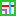).
3. In the ensuing dialog, check Ignore Existing Group Names and press .
1. In the lower center tab group, make the State Record pane active by clicking anywhere in the pane (if currently on top of the tab group) or on its tab (if currently “beneath” another pane).

2. Select the line

>>zone face skin slot-skin "Skin"

Right-click and choose Copy as Data File.

3. Make the tutorial data file active, set the cursor at the end of the file, and paste (Ctrl + V or Edit ‣ Paste).

4. Create groups for each of the five excavation stages. Type (or copy-paste from below) the lines that create the five groups (“exc1” through “exc5”) in the data file.

5. At the end of the data file, type (or copy-paste from below) model save "groups".

The commands from this step and step (6) that have been added to “tutorial.dat” should appear as below:

zone group "exc1" range position (0,  0,0) (1,0.8,2)
zone group "exc2" range position (0,0.8,0) (1,1.6,2)
zone group "exc3" range position (0,1.6,0) (1,2.4,2)
zone group "exc4" range position (0,2.4,0) (1,3.2,2)
zone group "exc5" range position (0,3.2,0) (1,4.0,2)


Note that these commands could also have been generated interactively using range selection tools in the Model pane. This was not done here for brevity.

6. Rerun the model to this point by pressing solve ().

Discussion

The Auto Assign Face Groups in the Model pane is a tool that identifies groups of contiguous zone faces based on a break angle (45ยบ by default) and creates automatically named zone face groups for them.  In this case, using the tool results in six face groups being created, one for each “side” of the problem’s cubic geometry.

Note something specific happens here: the model state is changed to include the newly created face groups. However, the command that caused this change is not a part of the “tutorial” data file. In order to ensure our data file is complete, we need to capture the command that created the groups. We use the State Record pane to do this. As its name implies, the State Record pane records any command (from a data file, from the command prompt, from the user interface) that changes the model state, as it is issued, and stores it in a record. The record therefore contains all commands necessary to create the current model state. As the object in this tutorial is to arrive at a single complete data file, we use the State Record pane to copy the command that was used to create the face groups with the Auto Assign Face Groups tool and paste it into our data file.

The zones in each of the five excavation stages are grouped as well using the zone group command. Here one of the principal advantages of putting objects into named groups is clear: the names assigned to groups can have a logical relationship to one another and to the rest of the model. When the commands that will be used to perform the excavation sequence appear later, the names of the groups will help clarify what is going on in the commands.

Once the logical parts of the model have been identified and put into groups, it is a good idea to save the model state (review :ref:-solutionasflac3dproject-). CS: this figure (or something similar) is still a good idea, but gone for now (see flac3d 6 doc set) The final line that is added at step (7) does this.

When to Group

Designating groups is not strictly necessary at this stage, but it is highly advisable, as construction of commands that use groups as range targets is usually faster, easier, and perhaps more innately sensible than constructing a range with the other range elements. Groups are also quite useful later on for plotting. Lastly, using groups helps conceptualize the model in terms of its meaningful parts. That said, the Model pane can be used for model object selection and grouping at any time in a modeling project.

See In the Interface for some additional information about the program response to the commands performed here. Some further discussion on groups follows in the topic Further Discussion: Model Groups.

### Further Discussion: Model Groups

In a sense, all objects in a model are not equal. Some represent important model constructs (e.g., boundaries, interfaces), some represent natural features (joints, seams, or beds), and some represent human constructions (tunnels, structural support). And others don’t. Groups are a way of identifying the model components that are a part of the former group, as they will usually become the focal point of the majority of commands that are used to construct the model.

Ranges and Groups, Briefly

As this tutorial provides a first look at the FLAC3D range logic and groups, a word to distinguish them may be of use. A range uses one or more criteria to identify the objects on which a command should operate—if a range is not supplied on a command, all possible target objects of the command will be affected (see How Commands Work). A group is what its name implies: a collection of specific objects going by a given group name.

The initial definition of a group is made by specifying a range that determines the member objects.

zone group "lower" range position-z 1 100


This creates a group of zones named “lower”. It is possible that after cycling, the zones that fall in that range z 1 100 will be different. The zones in the group “lower”, however, will be the same.

Once created, a group can be the target of a command by specifying it as the defining criterion of a range. Consider:

zone cmodel assign elastic range group "lower"


This assigns the Elastic model to the zones in the group “lower”.

### In the Interface

Click the Model pane to make it active (if it isn’t already). The model should appear similar to the image below.Zones that are created by any method—with the zone create command, or through the Extrusion or Building Block panes—are rendered in the Model pane.

This area is capable of allowing the user to interact with model elements—zones or zone faces at this stage of the process—via mouse-driven selection tools. Any area of the model that may be of specific interest can be selected and defined to be a group.

• The “Saved States” section of the Project pane now lists two .f3sav files. The active model state is “groups.f3sav” (the inactive model state listed here, “geom.f3sav”, is grayed out).
• The “Objects” control set in the Control Panel indicates what is rendered in the view. At any time, the zones in a model may be rendered as either zones or zone faces, but not both. For this reason, the item is shown as “Zone / Zone Faces”, with a box around the currently rendered item. Clicking the word “Zone” or “Zone Faces” will set the clicked item to be the one rendered in the pane. As an immediate visual feedback, when zones are being rendered, the faces are outlined in black. When Zone Faces are being rendered, the faces are outlined in white.
• Double-click the item “geom.f3sav” in the “Saved States” section of the Project pane and observe the result. Double-clicking restores the “geom” model state, and it activates the Project pane. The model disappears from view! Now reactivate the Model pane by clicking on it. Observe that currently “Zone Faces” are to be rendered, but looking at the “Attributes for Zone Face” area, it can be seen that the “Show” attribute for this item is set to “Skin”. There was nothing in the “Skin” slot when geom.f3sav was saved; therefore, there is nothing to show. Change the “Show” item to “Surface of Visible Zones”; this will make the model visible again.
• As “geom.f3sav” is the active state, all zone faces are rendered in a single color, because there are no face groups in this model state. Double-click “groups.f3sav” to restore that model state, then click in the Model pane to reactivate it. Now the model is displayed with its excavation groups visible. Check the “Color Labels” control set to identify the color assignments in the current rendering.
• To experiment, open the “Rendering” item in “Attributes for Zone Face” and observe the effect of changing the settings it contains.

Rendering in either the Model pane or in a View pane (the pane type for plots) follows the same basic principle, which is also explained in In the Interface following the Examine Model: Plotting topic.

• The items to be rendered in the view are listed in the upper section of the “Objects” (Model pane) or “Plot Item List” (View pane) control set in the Control Panel.
• With an object selected in the upper section, the lower section provides an “Attributes” set that supplies controls for setting how the selected object should be rendered.

## Specifying Models, Boundaries, and Initial Conditions

Next a series of commands is added to complete the initial model definition.

1. Type or copy-paste the lines below into the “tutorial” data file.

; assign constitutive model, initial, and boundary conditions
zone cmodel assign mohr-coulomb
zone property density 1000 bulk 1e8 ...
shear 3e8 friction 35 ...
cohesion 1e3 tension 1e3
zone face apply velocity-normal 0 range group "West"
zone face apply velocity-normal 0 range group "Bottom"
zone face apply velocity-normal 0 range group "North"
model gravity (0,10,0)
zone initialize-stresses ratio 0.5
zone face apply stress-normal 0 gradient (0,[-10*1000*0.5],0) ...
range group "East" or "Top"
; use of inline FISH in preceding line illustrates
; the derivation of the value needed (-5000)

; step to equilibrium
model large-strain true

2. Rerun the model to this point by pressing solve ().

Previous: Create Model Groups | Next: Step to Equilibrium

Discussion: Assigning a Constitutive Model

Once the grid generation and model partitioning (grouping) is complete, one or more material models and associated properties must be assigned to all zones in the model. These are done using the commands zone cmodel assign and zone property. Here, the zone cmodel assign command that assigns a mohr-coulomb model is supplied without a range. Consequently, it will be applied to all zones in the model.

Properties are set with zone property commands in a way that spans model assignments. Setting the bulk property without a range will set it for any zone whose assigned constitutive model uses a bulk property (and this is most of them). Limiting the specification of a property to zones with a particular constitutive model assignment is done by using a range that invokes the constitutive model assignment as a criterion in a range. For example zone property bulk 1e4 range model mohr-coulomb (contrast this to what is given above) would set the bulk property only for zones that have been assigned the mohr-coulomb model.

Lastly, note the introduction of inline FISH. The second term of the gradient vector is provided as a mathematical expression enclosed in brackets ([ ]). The brackets invoke the FISH language, which takes care of performing the calculation and returning the result.

See Further Discussion: Assigning a Constitutive Model for additional discussion of constitutive model assignment.

Discussion: Applying Boundary Conditions

The three zone face apply commands are used to set roller boundary conditions on the symmetry planes “West”, “North”, and “Bottom” sides of the model (the named groups from the preceding step come in handy here).

See Further Discussion: Applying Boundary Conditions for additional discussion of applying boundary conditions.

Discussion: Specify Initial Conditions

In this problem, the initial stress state is subjected to gravitational loading. This is added with the model gravity command.

By default, boundaries in FLAC3D are free of stress and are unconstrained. For this problem, all stresses ($$\sigma_{xx}$$, $$\sigma_{yy}$$, and $$\sigma_{zz}$$) are initialized with the single command zone initialize-stresses; it is possible to supply a separate stress for each component as well (cf. zone initialize stress xx).

Finally, we choose to apply a stress boundary to the far field using the zone face apply command; the boundary is set to match the changes due to gravity through use of the gradient keyword.

See Further Discussion: Specifying Initial Conditions for additional discussion of specifying initial conditions.

See In the Interface for some additional discussion of the steps taken here. Relative to this example problem, assigning constitutive models, applying boundary conditions, and specifying initial conditions are each described further in their own topics.

### Further Discussion: Assigning a Constitutive Model

Assigning a Constitutive Model

FLAC3D has nineteen built-in mechanical material models. (described in Section 1.2 in Constitutive Models) Three models are sufficient for most analyses the new user will make: null, elastic, and mohr-coulomb.

The null model represents material that is removed or excavated from a model. The elastic model assigns isotropic elastic material behavior. The mohr-coulomb model assigns Mohr-Coulomb plasticity behavior.

Models elastic and mohr-coulomb require that material properties be assigned via the zone property command. For the elastic model, the required properties are bulk and shear modulus, or Young’s modulus and Poisson’s ratio.

NOTE: Bulk modulus, $$K$$, and shear modulus, $$G$$, are related to Young’s modulus, $$E$$, and Poisson’s ratio, $$ν$$, by the following equations: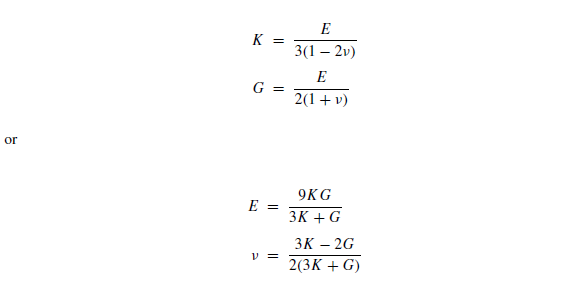The following are required properties for the Mohr-Coulomb plasticity model:

• bulk and shear moduli, or Young’s modulus and Poisson’s ratio;
• friction and dilation angles;
• cohesion; and
• tensile strength.

If any of these properties is not assigned, its value is set to zero by default.

Zones may be assigned different material models. For example, an elastic model may be prescribed for the upper half of a 10 m × 10 m × 10 m grid, and a Mohr-Coulomb model for the lower half of the grid. The example below shows how this is done using a range based on $$z$$-coordinates.

model new
zone create brick size 10,10,10
; elastic in upper half of grid
zone cmodel assign elastic range position-z=5,10
zone property bulk 1.5e8 ...
shear 0.3e8 ...
range position-z=5,10
; mohr-coulomb in lower half
zone cmodel assign mohr-coulomb range position-z=0,5
zone property bulk 1.5e8 ...
shear 0.6e8 ...
friction 30 ...
cohesion 5e6 ...
tension 8.66e6 ...
range position-z=0,5


### Further Discussion: Applying Boundary Conditions

Boundary conditions are normally specified with the zone apply or zone face apply commands; they are removed with corresponding zone apply-remove and zone face apply-remove commands.

The boundary conditions in a numerical model consist of the values of field variables that are prescribed at the boundary of the numerical grid. Note that by using a boundary condition command, while FLAC3D is calculating a solution, a condition or constraint is imposed that will not change unless specifically changed by the user. Boundaries can be either real or artificial: real boundaries exist in the physical object being modeled; artificial boundaries are introduced to reduce the size of the model.

Artificial boundaries fall into two categories: planes of symmetry and planes of truncation. A symmetry plane takes advantage of the fact that the geometry and loading in a system are symmetrical about one or more planes. A truncation plane is a boundary sufficiently far from the area of interest that the behavior in that area is not greatly affected. It is important to know how far away to place these boundaries and what errors they might precipitate in the stresses and displacements computed for the area of interest.

In this tutorial, we identify two planes of symmetry for the problem: one is the $$x$$$$y$$-plane; and the other is the $$y$$$$z$$-plane through the center of the trench. The commands that create this much of the model are as seen here.

model new
; Create zones
point 0 (0,0,0) point 1 (10,0,0) point 2 (0,10,0) point 3 (0,0,10) ...
size 3,5,5,7 ...
ratio 1,1,1,1.5 ...
dim 1 4 2 fill group 'exc'
zone face skin
; Constitutive model and properties
zone cmodel assign mohr-coulomb
zone property bulk 1e8 shear 3e8 friction 35 ...
cohesion 1e3 tension 1e3 density 1000
; Boundary conditions
zone face apply velocity-normal 0 range group 'West'
zone face apply velocity-normal 0 range group 'Bottom'
zone face apply velocity-normal 0 range group 'North'
zone face apply stress-normal 0 gradient (0,-5000,0) range group 'East'
zone face apply stress-normal 0 gradient (0,-5000,0) range group 'Top'
model gravity (0,10,0)
zone initialize-stresses ratio 0.5


This yields a quarter-symmetry model as shown below (compare this to the full geometry as shown in the figure in the topic Further Discussion: Meshing With Primitives).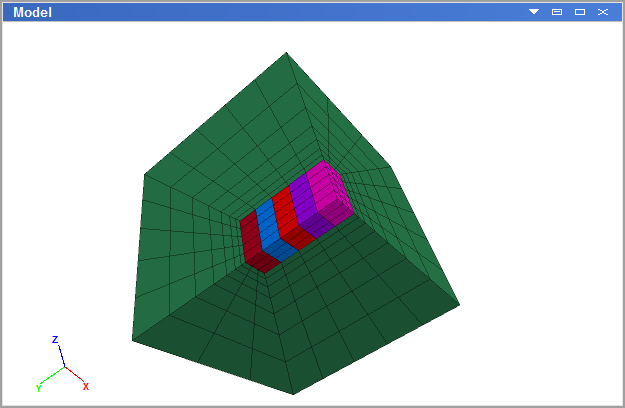Figure 5: Quarter-symmetry model of trench excavation.

By default, the boundaries in FLAC3D are free of stress and are unconstrained. Forces or stresses may be applied to any boundary, or portion of a boundary, by means of the zone apply or zone face apply command. Individual components of the stress tensor may be specified. For example, a constant, compressive $$x$$$$x$$-stress component of 10 MPa can be applied to a boundary located at $$x$$ = 10 with the command

zone face apply stress-xx -10e6 range position-x 10


Remember that compressive stresses are negative. The range keyword is used at the end of the command line to specify the range of action for the zone face apply command shown here. In this case, the boundary condition applies to any boundary faces that fall within the default tolerance (1e-7) of $$x$$ = 10. A linearly varying $$y$$$$y$$-stress component can be applied with the gradient keyword. For example,

zone face apply stress-yy -20e6 gradient (0,0,20e5) ...
range position-y 0 position-z 0 10


With this command, a $$yy$$-stress component is applied to boundary faces located at $$y$$ = 0. The $$y$$$$y$$-stress varies linearly with $$z$$ from $$σ_{yy}$$ = 0 at $$z$$ = 10, to $$σ_{yy}$$ = −20 × 106 at $$z$$ = 0.

When gradient is used, a value varies according to the relation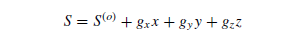in which $$S$$(^o ) is the value at the global coordinate origin at ($$x$$ = 0, $$y$$ = 0, $$z$$ = 0) and is the value immediately following the stress-syy keyword, and $$gx$$ , $$gy$$, and $$gz$$ specify the variation of the value in the $$x$$-, $$y$$-, and $$z$$-directions and are the three numbers following the gradient keyword.

Note

*Velocity has units of displacement per cycle or step for the static solution mode and displacement per unit time for the dynamic mode.

Displacements cannot be controlled directly in FLAC3D. In fact, they play no part in the calculation process. In order to apply a given displacement to a boundary, it is necessary to prescribe the boundary’s velocity for a given number of steps. If the desired displacement is $$D$$, a velocity $$V$$ over $$N$$ steps (where $$N$$ = $$D/V$$) may be applied.* In practice, $$V$$ should be kept small and $$N$$ large in order to minimize shocks to the system being modeled. The zone face apply command can be used to specify the velocities; gradients may also be specified.

In addition to gradient, the keywords add, multiply, and vary are available for use to modify the values of zone apply and zone face apply commands (and others).

The following command shows how to fix the $$x$$-displacement to zero along a boundary located at $$x$$ = 0:

zone face apply velocity-x 0 range position-x 0


This, in effect, creates a roller boundary at which the boundary plane is fixed in the $$x$$-direction, but free to move in the $$y$$- and $$z$$-directions.

A pinned boundary condition (i.e., constrained in the $$x$$-, $$y$$-, and $$z$$-directions) can be specified with the command

zone face apply velocity (0,0,0) range position-x 0


For real boundaries, the choice of stress or displacement boundary conditions is usually clear. However, for artificial boundaries, such as planes of truncation, either type may be selected. Experience has shown that, generally:

• a fixed boundary causes both stresses and displacements to be underestimated;
• a stress boundary causes both stresses and displacements to be overestimated; and
• the two types of boundary conditions bracket the true solution, so that it is possible to do tests with both boundaries and get a reasonable estimate of the true solution by averaging the two results.

### Further Discussion: Specifying Initial Conditions

Unlike the boundary condition commands, the initial condition commands assign initial values to selected variables; these can change while the computation proceeds. For example, in geological materials, there is an in-situ state of stress in the ground before any excavation or construction is started. By setting the initial conditions in a FLAC3D grid, an attempt is made to reproduce this in-situ state because it will influence the subsequent behavior of the model. (This influence is discussed in more detail in Boundary Conditions topic.)

To prescribe an initial stress state, we use the zone initialize command. For example,

zone initialize stress xx -50e6 yy -40e6 zz -10e6


This assigns initial compressive stress components of

$$\sigma_{xx}$$ = −50e6
$$\sigma_{yy}$$ = −40e6
$$\sigma_{zz}$$ = −10e6

to all zones in the model. All six stress components can be initialized (either individually or all together in one command), and a stress gradient can be specified for boundary stresses in the same manner as previously discussed in Further Discussion: Applying Boundary Conditions (see also Value Modifiers (add, multiply, gradient, & vary keywords) for usage).

If the initial stress state is subjected to gravitational loading, this may be added via the model gravity command. For example,

model gravity (0,-9.81,0)


in which a gravitational acceleration vector of 9.81 m/sec2 is applied in the negative $$y$$-direction. If gravitational loading is specified, the material mass density must also be initialized with the command zone initialize density.

In the example in this tutorial, $$σ_{xx}$$ and $$σ_{zz}$$ stresses and gradients are equal to half the $$σ_{yy}$$ stresses and gradients. Stresses may be initialized to any value, but you must check the stress state to ensure that it does not violate the yield criterion (Mohr-Coulomb, in this case). The initial equilibrium state is discussed further in the next section.

## Step to Equilibrium

Next a series of commands is added to complete the initial model definition.

1. Type or copy-paste the lines below into the “tutorial” data file.

model solve
model save "tr_eq"

; monitor trench excavation
zone gridpoint initialize displacement (0,0,0)

2. Rerun the model to this point by pressing solve ().

3. Make “Plot01” the active tab.

4. Press the Build Plot button (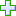) in the Control Panel, and double-click “History Chart”.

5. In the “Attributes” control set, press the plus button () adjacent to the label “mechanical ratio limit”.

6. In the attributes for the History item, open the “Y-Axis” group (press the arrow adjacent to the label) and check the “Log” box to set the $$y$$-axis of the plot to a logarithmic scale.

Discussion: Stepping to Equilibrium

The FLAC3D model must be at an initial force-equilibrium state before alterations can be performed. For simple model geometries, the boundary conditions and initial conditions may be assigned such that the model is exactly at equilibrium initially. However, in most cases, it is necessary to step the model to the initial equilibrium state under the given boundary and initial conditions, particularly for problems with complex geometries or multiple materials. This is done using either the model step (or its synonym model cycle) or model solve command.

In this example, before the model solve command is issued, the program is set to use large-strain calculation, which will move the position of the zones as displacements develop. Also, a history of average convergence ratio is set to be recorded when the model cycles. The history will be used as a means of visualizing and verifying that the model reaches equilibrium.

The model will cycle for 1805 steps when the model solve command is given. When complete, this is another juncture where saving the model state is advisable; the last command in the listing above does this.

To verify the model has reached equilibrium, we create a plot of the convergence ratio (steps (3)-(6) above do this). The resulting image should match the one below. The plot indicates that a ratio of 1e-5 is reached after 1805 steps.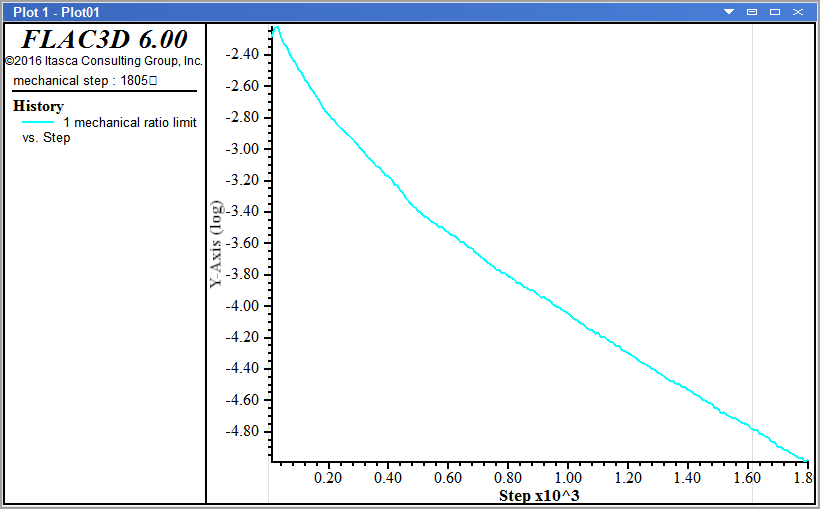Further introductory discussion of cycling to equilibrium follows in the topic Further Discussion: Step to Equilibrium.

Observations about the program response to the commands issued here is covered in the topic In the Interface.

### Further Discussion: Step to Equilibrium

A model is in perfect equilibrium when the net nodal-force vector at each gridpoint is zero (see Theoretical Background and following). The convergence criteria used is monitored in FLAC3D and printed to the screen when the model solve command is executing. In this way, the user can assess when equilibrium has been reached.

For a numerical analysis, the average force ratio will never reach exactly zero. It is sufficient, though, to say the model is in equilibrium when it reaches a small value. The default limit of 1e-5 is considered adequate in the majority of cases.

This is an important aspect of numerical problem-solving with FLAC3D. The user must decide when the model has reached equilibrium.

There are several features built into FLAC3D to assist in making this decision. The history of the maximum unbalanced force may be recorded by adding a history with the command

zone create brick


Additionally, the history of selected variables (e.g., velocity or displacement at a gridpoint) may be recorded. The following commands are examples:

model history mechanical ratio
zone history velocity-x position (3,4,4)


The first history command records $$x$$-velocity at location ($$x$$ = 3, $$y$$ = 4, $$z$$ = 4), while the second records $$z$$-displacement at location ($$x$$ = 0, $$y$$ = 0, $$z$$ = 8).

After running several hundred (or thousand) calculation steps, a history of these records may be plotted to indicate the equilibrium condition. The example below illustrates this process.

model new
model large-strain off
; Create zones
zone create brick size 6 8 8
zone face skin
; Assign constitutive model and properties
zone cmodel assign elastic
zone property bulk 1e8 shear 0.3e8
; Boundary Conditions
zone face apply velocity-normal 0 range group 'East' or 'West'
zone face apply velocity-normal 0 range group 'North' or 'South'
zone face apply velocity-normal 0 range group 'Bottom'
zone face apply stress-normal -1e6 range group 'Top'
; Histories
model history mechanical ratio
zone history velocity-x position (3,4,4)
zone history displacement-z position (0,0,8)
; Step
model step 1500
;model solve


The initial average force ratio maximum unbalanced force is about 0.19. After 1500 steps, this force has dropped to approximately .00002. By plotting the first history, it can be seen that the average force ratio is approaching zero. This can be done by creating a plot window, adding a “History” plot, and selecting the mechanical ratio limit history in the Attribute panel.

Following sentence is incomplete. at the command prompt in the Console pane. The result should appear as below.

The following figure is way bigger than the others.

The velocity also approaches zero, and the displacement history becomes constant; these are indicators that an equilibrium state is reached.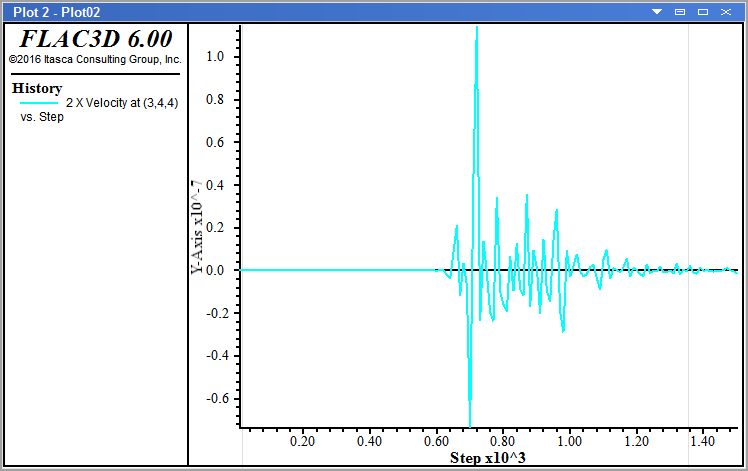Figure 7: x-velocity history at (x = 3, y = 4, z = 4).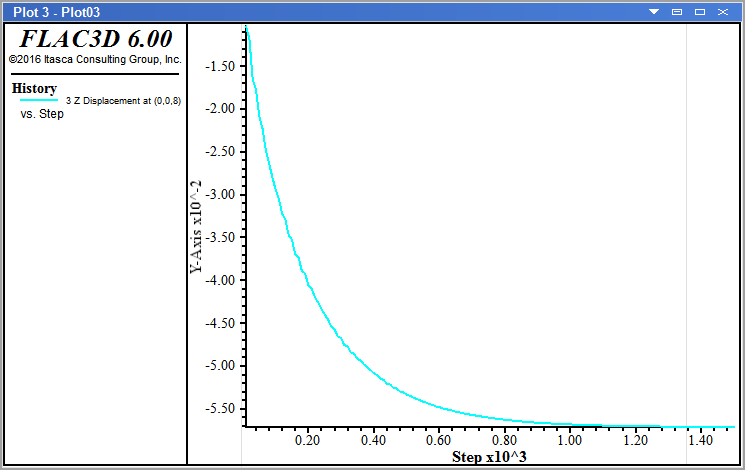Figure 8: z-displacement history at (x = 0, y = 0, z = 8).

The model solve command can be used in place of model step if the user wishes FLAC3D to stop automatically when the maximum unbalanced force falls below a specified limit. Uncomment (delete “;”) the command model solve and comment (add “;” in front of) model step 1500 and re-run the preceding problem. This time, FLAC3D should stop the calculation at step 1657. The plots (if created as previously shown) are automatically updated, though the results are essentially the same as shown above.

By default, an average force ratio limit of 1.0 × 10-5 is used if no other limits are specified in a model solve. The ratio can be changed by typing

zone face apply velocity (0,0,0) range position-z 0


before giving the model solve command. Here 1e-6 is a user-supplied value that sets the limit of the current convergence ratio.

In the example above, the initial equilibrium stage can be achieved without stepping by inserting a zone initialize stress command before the model solve command:

model solve convergence 0.1


Now, the average force ratio is nearly zero. After inserting the line above, press thebutton to re-run the problem. Note that, in this case, the initial displacements in the model are automatically zero. Looking back at the example shown in the topic Further Discussion: Applying Boundary Conditions, the model described by the data file should be at a stress equilibrium state. If you run that data file and then type in

zone initialize stress xx -1e6 yy -1e6 zz -1e6


at the command prompt, you will find that a small number of cycles is still required to reach the limit of 1 x 10-5. This is because of the graded mesh. If the zones were all of equal size, the unbalanced force would be nearly zero. However, with graded meshes, it is usually necessary to perform some stepping to bring the model to an initial equilibrium state.

In an analysis, it is very important that the model be at equilibrium before alterations are made. Several histories should be recorded throughout a model to ensure that a large force imbalance does not exist. It does not adversely affect the analysis if more steps than needed are taken to reach equilibrium. However, it will affect the analysis if too few steps are taken.

A FLAC3D calculation can be interrupted at any time during stepping by pressing <Shift+Esc>. It can be convenient to use the model step command with a high step-number to periodically interrupt the stepping, check the histories, and resume stepping until the equilibrium condition is reached.

### In the Interface

When cycling, there are some aspects of program operation that are worth noting.Figure 9: The “Cycling Info” dialog.

• The Cycling Info dialog displays a running total of the number of steps in the current step/cycle/solve command, the total number of cycles in the model, the average stress ratio, and the clock time for the current step/cycle/solve command.
• The current cycling may be interrupted by pressing the button or by pressing the Shift + Esc key.
• With regard to the tutorial, note the most recent saved state, “tr_eq.f3sav”, is added to the “Saved States” list in the Project pane (and it is the active state).
• Here and anywhere else it is encountered in FLAC3D, a Help button () will open a help file topic that provides contextual information for the current item.

## Alter Model: Excavation

Now the model excavations are performed.

1. Type or copy-paste the lines below into the “tutorial” data file.

zone history displacement-z position (.01,.01,2)

; excavation step 1
zone cmodel assign null range group "exc1"
model step 1000
model save "exc1"
; excavation step 2
zone cmodel assign null range group "exc2"
model step 1000
model save "exc2"
; excavation step 3
zone cmodel assign null range group "exc3"
model step 1000
model save "exc3"
; excavation step 4
zone cmodel assign null range group "exc4"
model step 1000
model save "exc4"
; excavation step 5
zone cmodel assign null range group "exc5"
model step 1000
model save "exc5"

; install cable support
model restore "exc2"

2. Rerun the model to this point by pressing solve ().

3. Make the “Plot01” tab active.

Previous: Step to Equilibrium | Next: Examine Model: Plotting

Discussion: Performing Alterations

Before performing the excavations, a couple of additional histories are set up—one for $$x$$-displacement at the gridpoint at (1,0,0), and one for $$z$$-displacement at the gridpoint at (0,0,2).

Excavation is a matter of first assigning the zones of the current excavation stage to the null model. The model is then cycled for 1000 steps, and then the model state is saved before moving on to the next excavation stage. Note the zone groups for the excavation stages that were created in Create Model Groups are used for the zone cmodel assign commands that perform the excavation.

Looking at “Plot01”, we see that the five excavation stages have been added to the history seen in the previous step (we see one + five minimum points in the chart). Significantly, we see that the model fails at the third stage—it does not reach/approach a convergence ratio of 1e-5 before the next excavation step begins.

At this point, it should be observed that the use of the model step command in this example is a bit unrealistic. It is used here to help illustrate the modeling process efficiently. However, in a more rigorous model, the command model solve would be used, and the failure in the third step would be evident—the model would simply continue to cycle. You can test this by substituting model solve for model step 1000 at the third excavation stage. The model will eventually stop cycling, but with an “Illegal geometry” error; it never reaches equilibrium. This is a common terminating point on models exhibiting continuous deformation. It indicates a zone in the model has deformed to a state where calculations can no longer be performed on it.The next modeling phase of this tutorial will involve installing cables as support after the second stage and observing the effect. However, before doing so, we will explore plotting to get a further look at the failure that occurs in the third stage.

Further introductory discussion of staged modeling is provided in the topic Further Discussion: Performing Alterations.

### Further Discussion: Performing Alterations

FLAC3D allows model conditions to be changed at any point in the solution process. These changes may take the following forms:

• excavation of material;
• change of material model or properties for any zone; and
• fix or free velocities for any gridpoint.

Note

*If moduli (bulk or shear) are changed in a model that is in equilibrium, no immediate effect will be observed because FLAC3D uses tangent, rather than secant, moduli.

In this tutorial, excavation is performed using the zone cmodel assign null command. Gridpoint loads can be applied at any gridpoint with the zone apply (force, force-x, etc.) command. Stress alterations can be made at model boundaries with the zone face apply command, as shown previously. Material models and properties* are changed with the zone cmodel assign and zone property commands. Gridpoint velocities are fixed or freed using the zone face apply command to set velocity to the desired value(s). It should be evident that several commands can be repeated to perform various model alterations.

If model zones contain a plasticity model (e.g., zone cmodel assign mohr-coulomb), it is possible that an alteration may be such that force equilibrium cannot be achieved. In other words, the unbalanced forces in part or all of the model cannot approach zero, in which case, the average force ratio will approach a constant nonzero value, indicating that steady-state flow of material is occurring (i.e., a portion/all of the model is failing).

See :ref:-t109_installingsupport- after the topic :ref:-t1_09support- in this tutorial for a simple example model that exhibits clearly identifiable failure. CS: this needs to be revisited later

## Examine Model: Plotting

The following steps illustrate the basic mechanics of plotting.

1. Select File ‣ Add New Plot… and name it “xdisp” in the ensuing dialog. Note the newly created empty plot is now the active pane.
2. Press the Build Plot button (), select History Chart and press in the ensuing dialog.
3. In the “Attributes” section on the Control Panel:
• add History 2 “X Displacement at …” by pressing the add button adjacent to its label ();
• open the “X-axis” item, uncheck “Auto” on “Minimum” and “Maximum”, and type “0” in for the minimum and “6000” in for the maximum; and
• open the “Y-axis” item, uncheck “Auto” on “Minimum”, and type in the “-0.008” for the minimum value.
4. Repeat steps 1-2 to create a History plot of $$z$$-displacement named “zdisp”.
5. In the “zdisp” plot, go to the “Attributes” section:
• add History 3 “Z Displacement at …” by pressing the add button adjacent to its label ();
• open the “X-axis” item, uncheck “Auto” on “Minimum” and “Maximum”, and type “0” in for the minimum and “6000” in for the maximum; and
• open the “Y-axis” item, uncheck “Auto” on “Minimum”, and type in the “-0.0038” for the minimum value.
6. Press Ctrl + Shift + N, and name the new plot “xdisp2”.
7. Press the Build Plot button (), select Zone and press in the ensuing dialog.
8. In the “Attributes” control set:
• select “Contour” on the “Color By” switch;
• select “Displacement” on the “Value” switch;
• select “Z” on the “Component” switch;
• open the “Contour” item, and check the “Reversed” box; and
• uncheck “Auto” on “Minimum,” and set the value of the minimum to “-0.0003”.
9. Now, with any of these three plots active, step through the saved model states in the Project pane by double-clicking each saved state. Start with the “tr_eq” state (initial equilibrium), then progress through the excavation stages (“exc1” through “exc5”).

Previous: Alter Model: Excavation | Next: Further Alterations: Support

Discussion: Observing Failure

To observe the failure as the excavation sequence progresses, we plot the history items that were defined prior to running the model (immediately after initial equilibrium had been established). History plots will, by default, automatically compute the maximum and minimum values for the scales on the $$x$$- and $$y$$-axis of the chart. This is efficient and time-saving when looking at that history at a single model state. However, when looking at the same thing across a series of model states, it makes comparison of one view to the next difficult, because the scale on either axis is likely to change from one state to the next. For this reason, when setting up the plots here to help visualize the failure that occurs starting in the third excavation stage, we “fix” the scales on the plots by turning the “Auto” attribute off. As a result, when we step through the saved states (step (9) above), the fixed scales allow us to clearly see the failure develop without any “jumping around” in the chart.

Plots As Project Entities

Unlike command input or model states, plots are not stored separately as files. Once created, they will be saved with the project. Closing the pane containing a plot () eliminates it completely; it would need to be rebuilt to be returned. Plots can be hidden (press () on its pane) as needed; a hidden plot will still be listed on—and is re-accessed from—the “Documents” menu.

Four reference plots are named. When creating a new plot, FLAC3D will automatically suggest a numbered name (e.g., “Plot04”), but as can be seen here, providing a more descriptive name is helpful, especially when many plots have been created.Further discussion of the mechanics of plotting and some general conventions in FLAC3D are provided in the topic In the Interface.

### In the Interface

The basic mechanics of model visualization in the Model pane or in a plot in a View pane are as follows.

1. Put objects into the view. In the Model pane, this occurs automatically as model objects (zones, structural elements) are created. In a View pane, this is done by picking items from the Build Plot dialog using the Build Plot tool ().
2. The objects that are currently rendered in the view are listed above in the Control Panel.
3. To control how an object is rendered, select it on the list above. This will activate the controls for visualization that appear below—for that object—in the “Attributes” section.Figure 10: Two views of the Control Panel: the “Objects” control set in the Model pane (left), and the “Plot Item List” in a View pane.

• In the Model pane, Zones and Zone Faces are listed on the same line of the “Objects” control set. Only one may be rendered at a time. A box indicates which is currently being rendered. Clicking the other item’s label will cause it to be rendered instead.
• Selecting a plot item in a View pane causes a menu selector icon (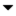) to appear to the right of the item name. The menu provides options to set attributes, add a plot item, or to cut, copy, delete, or paste the current item.

## Further Alterations: Support

Support is installed after the second excavation stage to stablize the trench and prevent the failure seen in the third excavation stage.

1. Type or copy-paste the lines below into the “tutorial” data file.

; install cable support
model restore "exc2"
structure cable create ...
by-ray (1.0,0.4,1.5) (1,0,0) 4 segments 4
structure cable create ...
by-ray (1.0,0.4,0.5) (1,0,0) 4 segments 4
structure cable create ...
by-ray (1.0,1.2,1.5) (1,0,0) 4 segments 4
structure cable create ...
by-ray (1.0,1.2,0.5) (1,0,0) 4 segments 4
structure cable property young 2e9 ...
yield-tension 1e8 ...
cross-sectional-area 1.0 ...
grout-cohesion 1e10 ...
grout-stiffness 2e9 ...
grout-perimeter 1.0
zone cmodel assign null range group "exc3"
model step 1000
model save "cab3"
structure cable create ...
by-ray (1.0,2.0,1.5) (1,0,0) 4 segments 4
structure cable create ...
by-ray (1.0,2.0,0.5) (1,0,0) 4 segments 4
structure cable property young 2e9 ...
yield-tension 1e8 ...
cross-sectional-area 1.0 ...
grout-cohesion 1e10 ...
grout-stiffness 2e9 ...
grout-perimeter 1.0
zone cmodel assign null range group "exc4"
model step 1000
model save "cab4"
structure cable create ...
by-ray (1.0,2.8,1.5) (1,0,0) 4 segments 4
structure cable create ...
by-ray (1.0,2.8,0.5) (1,0,0) 4 segments 4
structure cable property young 2e9 ...
yield-tension 1e8 ...
cross-sectional-area 1.0 ...
grout-cohesion 1e10 ...
grout-stiffness 2e9 ...
grout-perimeter 1.0
zone cmodel assign null range group "exc5"
model step 1000
model save "cab5"

2. Rerun the model to this point by pressing solve ().

Previous: Examine Model: Plotting | Next: Project Finishing

Discussion: Installing Cables

We return to the model state after two excavation stages, while it is still stable, by using the model restore command to put the model at that point. Now we add support in addition to nulling the zones for each excavation stage. This is done by spatially defining the cable, and then defining its properties. Then (as before) the excavation stage zones are nulled, the model is cycled 1000 steps, and the model state is saved. This sequence is repeated to create a new third, fourth, and fifth excavation stage.

### Further Discussion: Using Save/Restore and Staged Modeling

At this point, the function of the commands model save and model restore in a staged analysis should be apparent. At the end of a modeling stage (e.g., initial equilibrium), the model state can and should be saved using model save. This file can be restored at a later time using model restore, which will put the model back to the point at which the model was saved. Consequently, it is not necessary to build a model from scratch every time a change is made; simply save the model before the change and restore it to that point whenever a new change is to be analyzed.

For example, in this tutorial trench problem, the trench is excavated in 2 m deep stages. The model state is saved at the end of each stage, beginning with the initial equilibrium stage (“tr eq”). Looking at each stage in sequence, we can then determine the stage at which the trench fails, and investigate the influence of support (e.g., tiebacks or soil nails) on stabilizing the trench. For comparison, below we look at a model that excavates the trench all at once.

When we determine, upon running the model, that the trench fails at the third excavation stage, we simply restart the save file at the second excavation stage (exc2.f3sav), install cables, and then proceed with the excavation. This time, the trench walls are stable.

By using save files this way, we can perform a series of different calculations, starting from whatever stage we desire, and investigate various problem conditions, such as spacing and properties of cable elements.

Simpler Example

To illustrate the value of breaking the modeling process into stages, we can take the tutorial problem and simplify it. If we were to set up the model such that the entire trench is excavated at once, we might have a file that appears as below.

model new
; Create zones
point 0 (0,0,0) point 1 (10,0,0) ...
point 2 (0,10,0) point 3 (0,0,10) ...
size 3,5,5,7 ...
ratio 1,1,1,1.5 ...
dim 1 4 2 fill group 'exc'
zone face skin
; Assign constitutive model and properties
zone cmodel assign mohr-coulomb
zone property bulk 1e8 shear 3e8 friction 35 cohesion 1e3 ...
tension 1e3 density 1000
; Bondary condition
zone face apply velocity-normal 0 range group 'West'
zone face apply velocity-normal 0 range group 'North'
zone face apply velocity-normal 0 range group 'Bottom'
zone face apply stress-normal 0 gradient (0,-5000,0) ...
range group 'East' or 'Top'
; Initial conditions
model gravity (0,10,0)
zone initialize-stresses ratio 0.5
; Histories
zone history displacement-x position 1,0,0
; Excavation and setup
model large-strain on
zone relax excavate range group 'exc'
; ... and then calculate the response:
model step 1000


The displacement contours indicate the trench fails.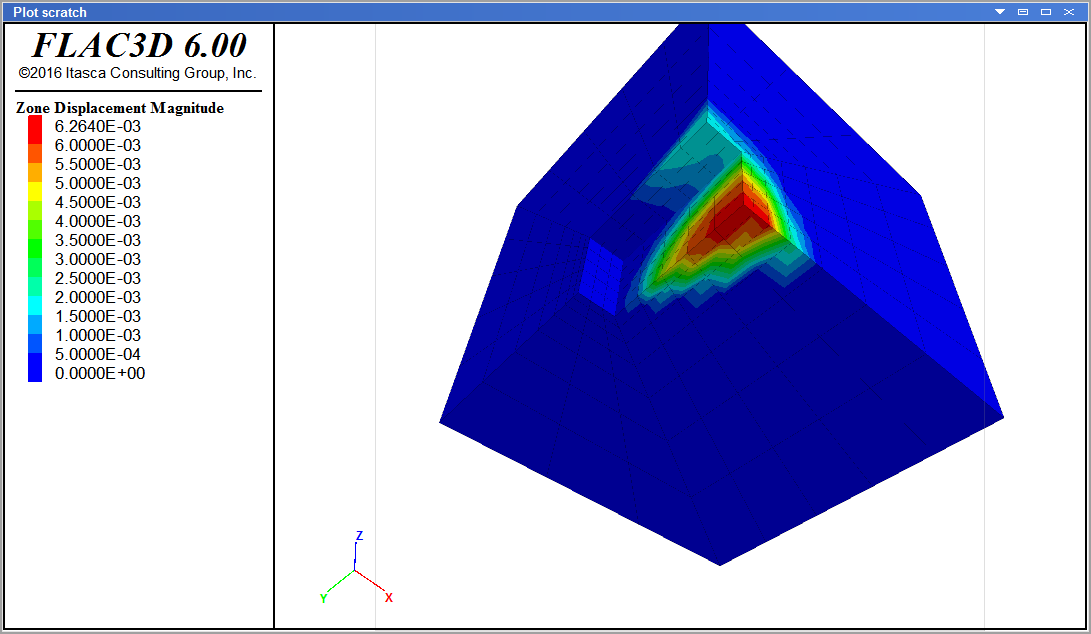The failure can also be indicated by plotting the displacement history at the trench wall.

The continuously increasing displacement indicates failure.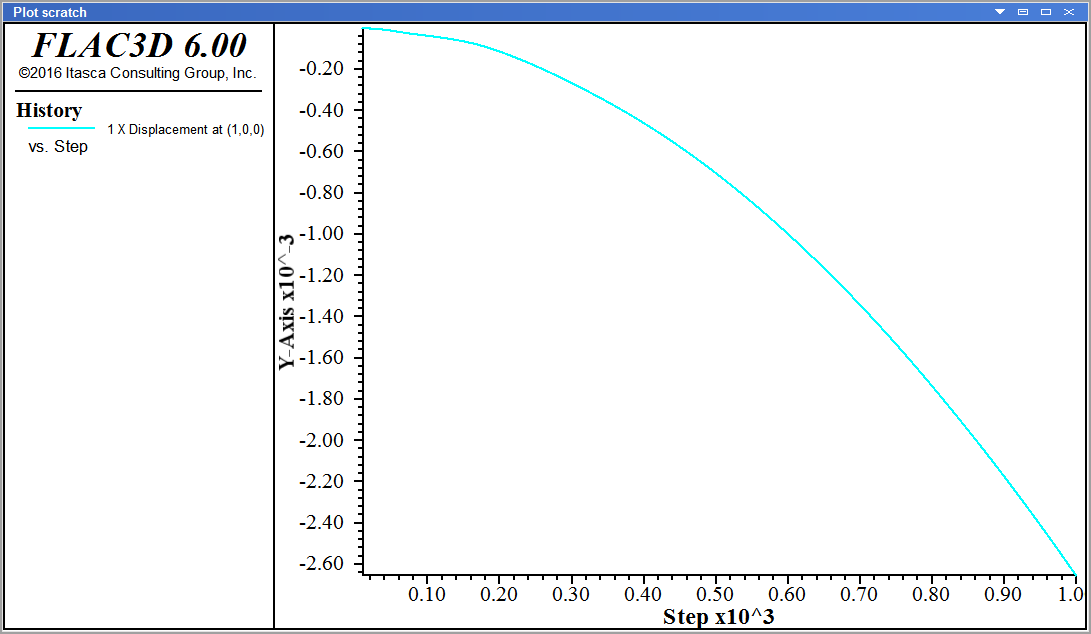You can see how this might be an early “draft” of the tutorial problem. Given this as a starting point, and given that failure is observed, a natural next step would be to look at model behavior when excavation is split up into a sequence, as is seen in the tutorial model. More precisely locating the point of failure in the model, in turn, provides an indiator of where it will be necessary to introduce support for the excavation.

## Project Finishing

The project is complete at this point. For the purposes of good management, a few additional steps are advisable.

1. Use File ‣ Save Project to save the current project.
2. Bundle the project by selecting the menu command Tools ‣ Bundle ‣ Pack…
3. In the ensuing dialog, review the contents to be packed, press , and provide a name for the bundle file.

Previous: Further Alterations: Support

Discussion: Project Completion

With the model runs completed, the project is saved and, for good measure, a bundle file is created for the project.

At this point, the modeling process, as shown in the General Solution Procedure, Illustrated topic , and how to accomplish that process in the |flac3d| program, as shown in :ref:-solutionasflac3dproject- topic, CS: see previous flag about this removed item should be clearer. This tutorial illustrates that model setup, and initial definitions tend to follow a singular path; however, once past the equilibrium stage, the potential for branching and bifurcation due to exploration, revision, and alteration is nearly limitless. In that context, a well-designed project with distinct stages is critically important.

A bundle is a compressed archive file that gathers up the following:

• any files input in the model (the files listed in the “Data Files” section of the Project pane);
• state record files (a record file corresponding to each save file listed in the “Saved States” section of the Project pane); and
• the project file

With a bundle file, all materials necessary to re-create the project are stored in a single location. Because the bundle file does not store save files, which can be quite sizeable when a model is large, it is a useful, efficient way to archive a project. The trade-off for that efficiency, however, is that using a bundle to re-create a project will require re-running the component data files or replaying the state record files in order to regenerate the project saved state(s).

Bundles are also an excellent way of attaching the project to a technical support request. This can be done automatically when requesting technical support via the technical support dialog (Help ‣ Request Technical Support…).

### In the Interface: Finishing• The bundle file report “previews” the content that will be included in the bundle.
• There should be a .record file corresponding to each .f3sav file in the project.
• The project file and all input files (data files, etc.) are included in the bundle.
• The file report categorizes the bundle contents in different sections: “OK”, “Warning: Files Modified But Not Saved”, “Error: Files Cannot Be Located (Link Broken)”, etc. For this tutorial, which is comparatively simple, all content is “OK”. Refer to the Bundle Report topic for reference.

Endnotes

  The file created in this tutorial is supplied with the program, so if the user prefers, the file can simply be loaded and the tutorial can be followed without building the file. The file is available with the program examples (from the Help menu, choose Examples…. In the ensuing dialog, pick FLAC -> UsersGuide -> Tutorial -> Illustrative -> FullTutorial.prj).
  It is possible to override the use of the project file’s folder as the current working directory using the program directory custom command. It is also possible to save a file from the command prompt to a folder other than the project folder by supplying an explicit path.
  If you want to run the examples shown on this page, there are two approaches you can take. Load the files: Each file shown on this page is supplied with the program. You can open the file into the tutorial project by using the menu command File ‣ Open Into Project… (or press Ctrl + O). The files are located in the current user’s application data area “application data area” should be a link to where the copy app data dialog is explained in the folder: [appdata location]\datafiles\UsersGuide\Tutorial\Illustrative The files shown on this page, in the order they appear, are: simple-brick.dat using-actual-coordinates.dat sloping-surface.dat regular-radial.dat combining-gen.dat reflecting.dat Build the files: You can copy and paste the examples shown on this page into a new data file(s). Open a data file for each example, or paste each into a single data file. If you choose the latter approach, to run a specific example you should select the lines of the example and press the “Execute Selected” button (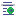) on the toolbar. All the examples begin with a model new command, which will clear the tutorial example model (or any other preceding example) before the current example is run.
  The Ignore Existing Names checkbox is used to ensure that the groups created are named without reference to existing groups. If not checked, then new group names will be formed as a concatenation of any pre-existing group names and the ones automatically generated during the processing of the zone face skin command.
  If you want to run the example above: Load the file: This file is “different-model.dat” in the folder [appdata location]\datafiles\UsersGuide\Tutorial\Illustrative Use the menu command File ‣ Open Into Project… (or press Ctrl + O) to open it. Build the file: Copy and paste the examples shown on this page into a new data file(s). Use Add New Data File… (or press Ctrl + N) to open a blank new data file.
  If you want to run the example above: Load the file: This file is “quarter-symmetry-trench.dat” in the folder [appdata location]\datafiles\UsersGuide\Tutorial\Illustrative Use the menu command File ‣ Open Into Project… (or press Ctrl + O) to open it. Build the file: Copy and paste the examples shown on this page into a new data file(s). Use Add New Data File… (or press Ctrl + N) to open a blank new data file.
  If you want to run the example above: Load the file: This file is “stepping-to-equilibrium.dat” in the folder [appdata location]\datafiles\UsersGuide\Tutorial\Illustrative Use the menu command File ‣ Open Into Project… (or press Ctrl + O) to open it. Build the file: Copy and paste the examples shown on this page into a new data file(s). Use Add New Data File… (or press Ctrl + N) to open a blank new data file.